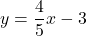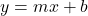## What is the slope of the line represented by the equation y = 4/5x – 3? A. -3 B. -4/5 C. 4/5 D. 3

Question

What is the slope of the line represented by the equation y = 4/5x – 3?
A. -3
B. -4/5
C. 4/5
D. 3

in progress 0
6 months 2021-07-20T12:35:19+00:00 2 Answers 19 views 0

## Answers ( )

1. C. 4/5 whichever number is with the x is the slope (y=mx+b)

C

Step-by-step explanation:

We have the equation:This is in slope-intercept form:Where m is the slope and b is our y-intercept.

Therefore, whatever number in front of our x, the coefficient, is our slope.

The coefficient of x is 4/5.

Therefore, the slope of the line is 4/5.

Hence, our answer is C.